# 干货推荐 | 掌握这几点，轻松玩转 Bokeh 可视化 （项目实战经验分享）

Bokeh recommend

作者 : Will Koehrsen



1. Bokeh 基础介绍
2. 在 Bokeh 中添加主动交互功能
3. 在 Bokeh 中创建交互式可视化应用程序

Tips：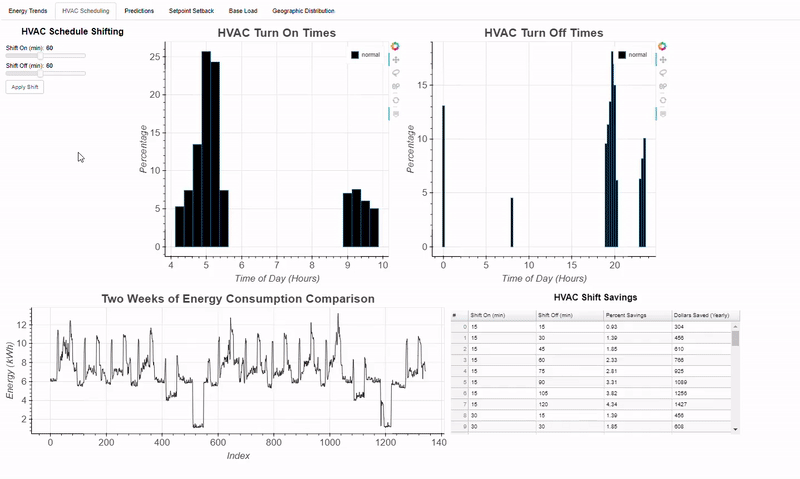## Bokeh 基础

Bokeh 的主要概念是图形一次构建一层。 我们首先创建一个图形(figure)，然后在图形中添加称为 图形符号(glyphs) 的元素。 glyphs 可以根据所需的用途呈现多种形状：圆形(circles)，线条(lines) ，补丁(patches)，条形(bars)，弧形(arcs)等。 让我们通过制作带有正方形和圆形的基本图表来说明 glyphs 的概念。 首先，我们使用 figure 方法创建一个图，然后通过调用适当的方法并传入数据将我们的 glyphs 附加到 figure 中。 最后，我们展示了所做的图表。

# bokeh basics
from bokeh.plotting import figure
from bokeh.io import show, output_notebook

# Create a blank figure with labels
p = figure(plot_width = 600, plot_height = 600,
title = 'Example Glyphs',
x_axis_label = 'X', y_axis_label = 'Y')

# Example data
squares_x = [1, 3, 4, 5, 8]
squares_y = [8, 7, 3, 1, 10]
circles_x = [9, 12, 4, 3, 15]
circles_y = [8, 4, 11, 6, 10]

p.square(squares_x, squares_y, size = 12, color = 'navy', alpha = 0.6)
p.circle(circles_x, circles_y, size = 12, color = 'red')

# Set to output the plot in the notebook
output_notebook()
# Show the plot
show(p)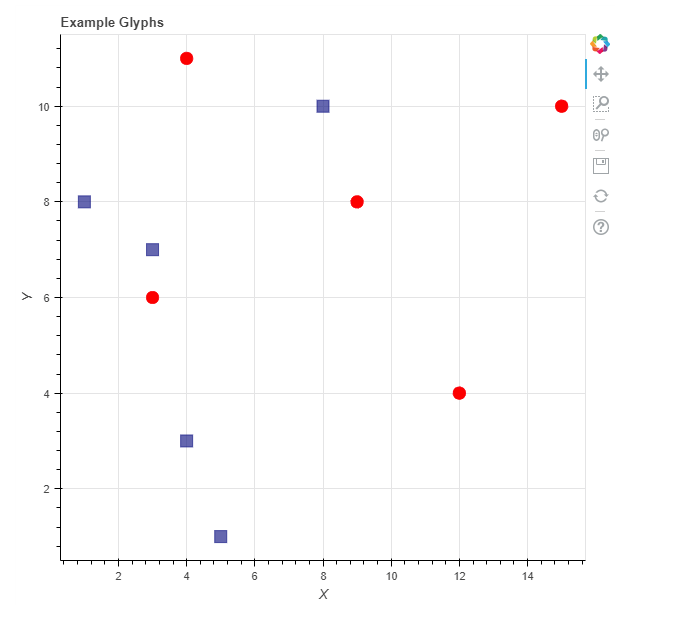# Read the data from a csv into a dataframe
# Summary stats for the column of interest
flights['arr_delay'].describe()

out[]:
count    327346.000000
mean          6.895377
std          44.633292
min         -86.000000
25%         -17.000000
50%          -5.000000
75%          14.000000
max        1272.000000



"""Bins will be five minutes in width, so the number of bins
is (length of interval / 5). Limit delays to [-60, +120] minutes using the range."""
arr_hist, edges = np.histogram(flights['arr_delay'],
bins = int(180/5),
range = [-60, 120])
# Put the information in a dataframe
delays = pd.DataFrame({'arr_delay': arr_hist,
'left': edges[:-1],
'right': edges[1:]})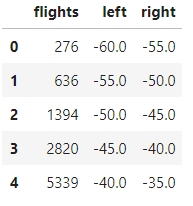flights 列是从 leftright 的每个延迟间隔内的航班数量。 从这里开始，我们可以创建一个新的 Bokeh 图形，并添加一个指定适当参数的 quad

# Create the blank plot
p = figure(plot_height = 600, plot_width = 600,
title = 'Histogram of Arrival Delays',
x_axis_label = 'Delay (min)]',
y_axis_label = 'Number of Flights')

left=delays['left'], right=delays['right'],
fill_color='red', line_color='black')

# Show the plot
show(p)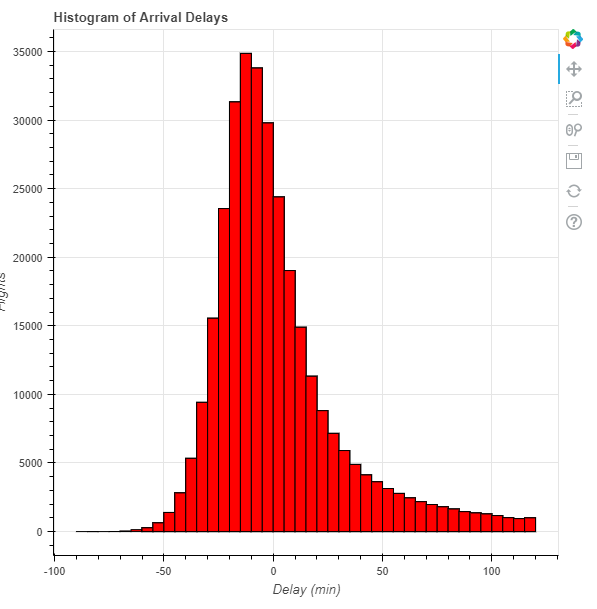### 增加交互性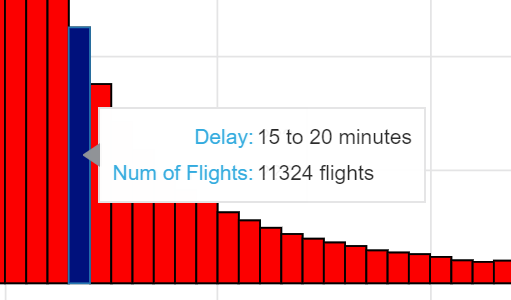# Import the ColumnDataSource class
from bokeh.models import ColumnDataSource
# Convert dataframe to column data source
src = ColumnDataSource(delays)
src.data.keys()

out:
dict_keys(['flights', 'left', 'right', 'index'])



# Add a quad glyph with source this time
left='left', right='right',
fill_color='red', line_color='black')



### Bokeh 中的 HoverTool

HoverTool 的语法起初可能看起来有些复杂，但通过练习它们很容易创建。 我们将 HoverTool 实例作为 Python 元组的 “tooltips” 列表传递，其中第一个元素是数据的标签，第二个元素引用我们想要突出显示的特定数据。 我们可以使用 $ 引用图表的任一属性，例如 x 或 y 位置，或使用 @ 引用我们数据源中的特定字段。 这可能听起来有点令人困惑，所以这里有一个 HoverTool 的例子： # Hover tool referring to our own data field using @ and # a position on the graph using$
h = HoverTool(tooltips = [('Delay Interval Left ', '@left'),
('(x,y)', '($x,$y)')])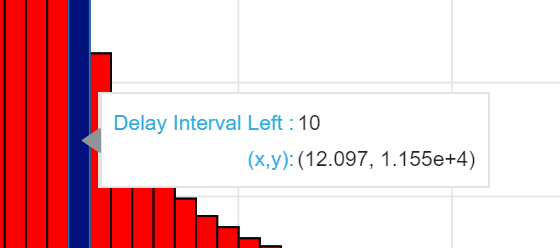（x，y）位置是图表上鼠标的位置，对我们的直方图不是很有帮助，因为我们要找到给定条形中对应于条形顶部的航班数量。 为了解决这个问题，我们将改变我们的 tooltip 实例以引用正确的列。 格式化提示工具中显示的数据可能令人沮丧，因此我通常在 dataframe 中使用正确的格式创建另一列。 例如，如果我希望我的提示工具显示给定栏的整个间隔，我在 dataframe 中创建一个格式化的列：

# Add a column showing the extent of each interval
delays['f_interval'] = ['%d to %d minutes' % (left, right) for left, right in zip(delays['left'], delays['right'])]



# Create the blank plot
p = figure(plot_height = 600, plot_width = 600,
title = 'Histogram of Arrival Delays',
x_axis_label = 'Delay (min)]',
y_axis_label = 'Number of Flights')

fill_color='red', line_color='black', fill_alpha = 0.75,
hover_fill_alpha = 1.0, hover_fill_color = 'navy')

# Add a hover tool referring to the formatted columns
hover = HoverTool(tooltips = [('Delay', '@f_interval'),
('Num of Flights', '@f_flights')])

# Style the plot
p = style(p)

# Add the hover tool to the graph

# Show the plot
show(p)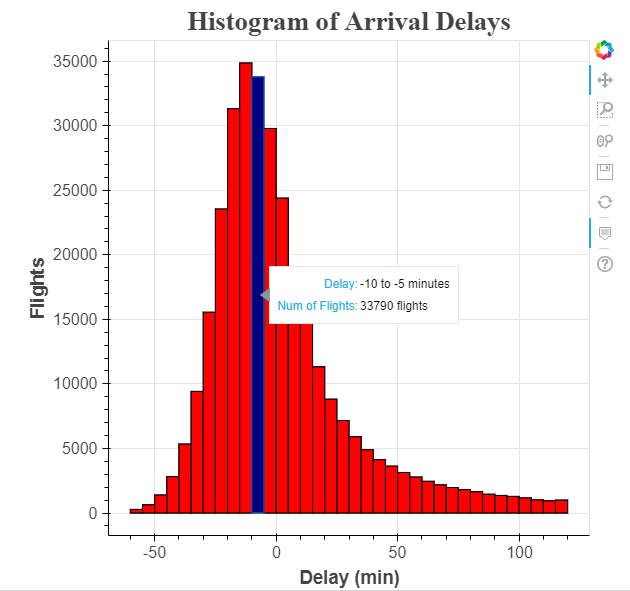# Import savings function
from bokeh.io import output_file
# Specify the output file and save
output_file('hist.html')
show(p)



## 在 Bokeh 中添加主动交互

Bokeh中有两类交互：被动交互和主动交互。 前面介绍的被动交互也称为检查器（inspectors），因为它们允许用户更详细地查阅图表中的信息，但不会更改显示的信息。 一个示例是当用户将鼠标悬停在数据点上时显示的提示信息，如下：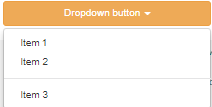### 主动互动的实现方法

• make_dataset(): 按特定格式整理要显示的特定数据
• make_plot(): 使用指定的数据绘图
• update(): 根据用户选择更新绘图

#### 整理数据

1. 航空公司 （在代码中称为 carriers）
2. 延迟的时间范围，比如： -60 至 +120 分钟
3. 直方图的宽度（即 bin 大小），默认值为 5 分钟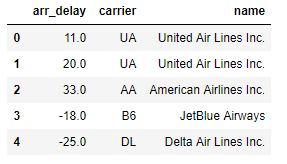def make_dataset(carrier_list, range_start = -60, range_end = 120, bin_width = 5):

# Check to make sure the start is less than the end!
assert range_start < range_end, "Start must be less than end!"
by_carrier = pd.DataFrame(columns=['proportion', 'left', 'right',
'f_proportion', 'f_interval',
'name', 'color'])
range_extent = range_end - range_start
# Iterate through all the carriers
for i, carrier_name in enumerate(carrier_list):

# Subset to the carrier
subset = flights[flights['name'] == carrier_name]

# Create a histogram with specified bins and range
arr_hist, edges = np.histogram(subset['arr_delay'],
bins = int(range_extent / bin_width),
range = [range_start, range_end])

# Divide the counts by the total to get a proportion and create df
arr_df = pd.DataFrame({'proportion': arr_hist / np.sum(arr_hist),
'left': edges[:-1], 'right': edges[1:] })

# Format the proportion
arr_df['f_proportion'] = ['%0.5f' % proportion for proportion in arr_df['proportion']]

# Format the interval
arr_df['f_interval'] = ['%d to %d minutes' % (left, right) for left,
right in zip(arr_df['left'], arr_df['right'])]

# Assign the carrier for labels
arr_df['name'] = carrier_name

# Color each carrier differently
arr_df['color'] = Category20_16[i]

# Add to the overall dataframe
by_carrier = by_carrier.append(arr_df)

# Overall dataframe
by_carrier = by_carrier.sort_values(['name', 'left'])
# Convert dataframe to column data source
return ColumnDataSource(by_carrier)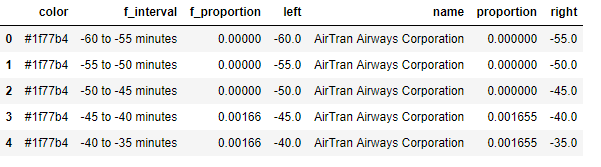def make_plot(src):
# Blank plot with correct labels
p = figure(plot_width = 700, plot_height = 700,
title = 'Histogram of Arrival Delays by Carrier',
x_axis_label = 'Delay (min)', y_axis_label = 'Proportion')

# Quad glyphs to create a histogram
p.quad(source = src, bottom = 0, top = 'proportion', left = 'left', right = 'right',
color = 'color', fill_alpha = 0.7, hover_fill_color = 'color', legend = 'name',
hover_fill_alpha = 1.0, line_color = 'black')

# Hover tool with vline mode
hover = HoverTool(tooltips=[('Carrier', '@name'),
('Delay', '@f_interval'),
('Proportion', '@f_proportion')],
mode='vline')

# Styling
p = style(p)

return p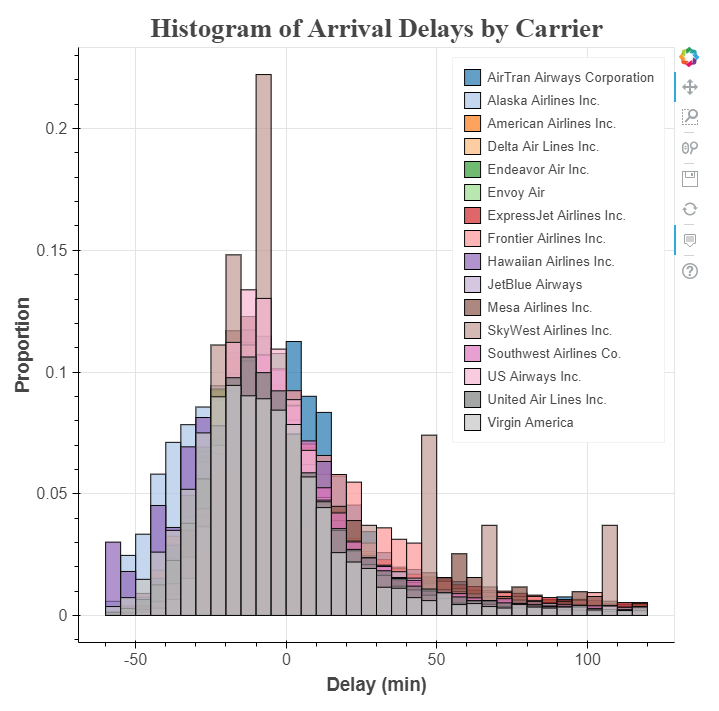#### 创建交互的小部件

from bokeh.models.widgets import CheckboxGroup
# Create the checkbox selection element, available carriers is a
# list of all airlines in the data
carrier_selection = CheckboxGroup(labels=available_carriers,
active = [0, 1])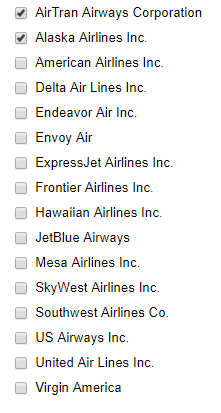Bokeh 复选框中的标签必须是字符串，而活动值是整数。 这意味着在图形中 ‘AirTran Airways Corporation’ 对应数字 0 ，’Alaska Airlines Inc.’ 对应数值 1。 当想要将所选复选框与航空公司匹配时，需要确保查找与所选整数活动值关联的字符串名称。 我们可以使用小部件的 .labels.active 属性来做到这一点：

# Select the airlines names from the selection values
[carrier_selection.labels[i] for i in carrier_selection.active]

out:
['AirTran Airways Corporation', 'Alaska Airlines Inc.']



# Update function takes three default parameters
def update(attr, old, new):
# Get the list of carriers for the graph
carriers_to_plot = [carrier_selection.labels[i] for i in carrier_selection.active]
# Make a new dataset based on the selected carriers and the
# make_dataset function defined earlier
new_src = make_dataset(carriers_to_plot,
range_start = -60,
range_end = 120,
bin_width = 5)
# Update the source used in the quad glpyhs
src.data.update(new_src.data)


# Link a change in selected buttons to the update function
carrier_selection.on_change('active', update)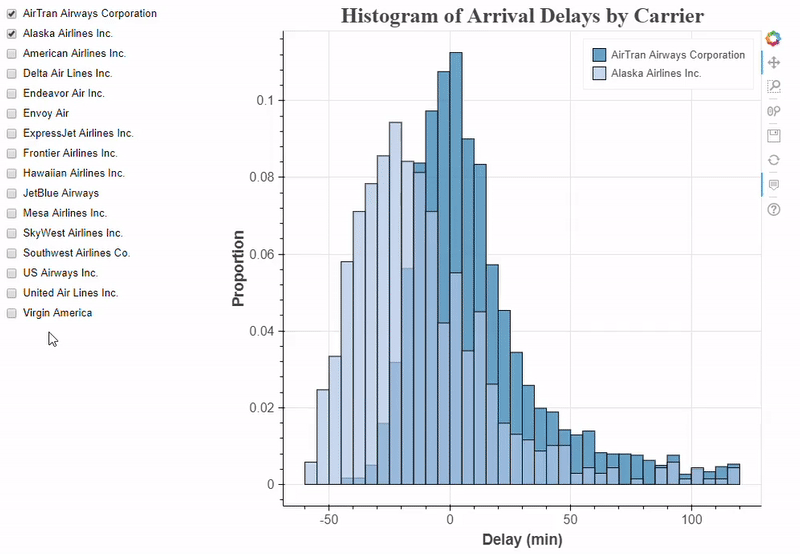### 更多的交互式控制

# Slider to select the binwidth, value is selected number
binwidth_select = Slider(start = 1, end = 30,
step = 1, value = 5,
title = 'Delay Width (min)')
# Update the plot when the value is changed
binwidth_select.on_change('value', update)

# RangeSlider to change the maximum and minimum values on histogram
range_select = RangeSlider(start = -60, end = 180, value = (-60, 120),
step = 5, title = 'Delay Range (min)')

# Update the plot when the value is changed
range_select.on_change('value', update)

# Update function that accounts for all 3 controls
def update(attr, old, new):

# Find the selected carriers
carriers_to_plot = [carrier_selection.labels[i] for i in carrier_selection.active]

# Change binwidth to selected value
bin_width = binwidth_select.value

# Value for the range slider is a tuple (start, end)
range_start = range_select.value
range_end = range_select.value

# Create new ColumnDataSource
new_src = make_dataset(carriers_to_plot,
range_start = range_start,
range_end = range_end,
bin_width = bin_width)

# Update the data on the plot
src.data.update(new_src.data)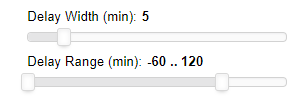# Change plot title to match selection
bin_width = binwidth_select.value
p.title.text = 'Delays with %d Minute Bin Width' % bin_width



### 把它们放在一起

from bokeh.layouts import column, row, WidgetBox
from bokeh.models import Panel
from bokeh.models.widgets import Tabs
# Put controls in a single element
controls = WidgetBox(carrier_selection, binwidth_select, range_select)

# Create a row layout
layout = row(controls, p)

# Make a tab with the layout
tab = Panel(child=layout, title = 'Delay Histogram')
tabs = Tabs(tabs=[tab])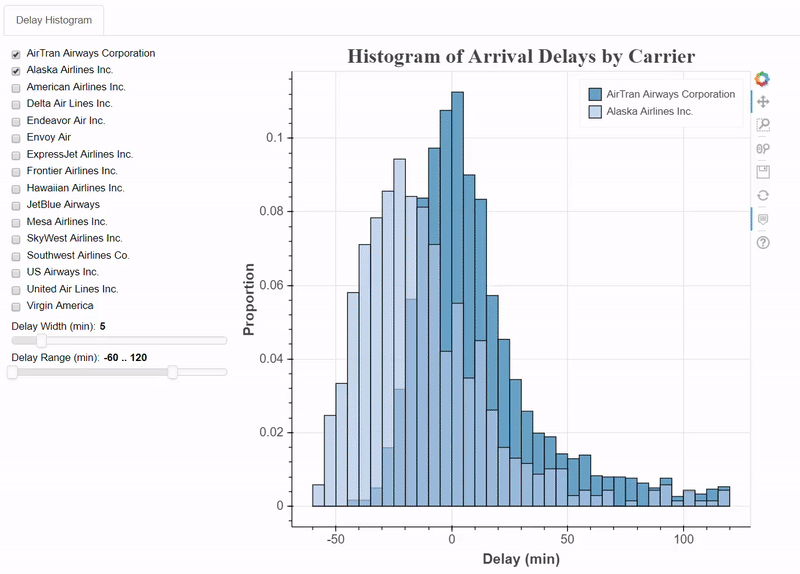## 在 Bokeh 中创建交互式可视化应用程序

### Bokeh 应用程序的文件结构

bokeh_app
|
+--- data
|   +--- info.csv
|   +--- info2.csv
|
+--- scripts
|   +--- plot.py
|   +--- plot2.py
|
+--- main.py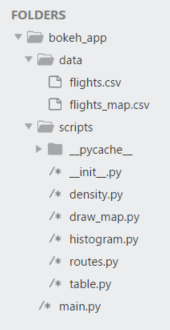### 主程序文件 （main.py）

main.py 脚本就像一个 Bokeh 应用程序的执行程序。 它加载数据，将其传递给其他脚本，返回结果图，并将它们组织到一个显示中。 这将是我完整展示的唯一脚本，因为它对应用程序尤其重要。

# Pandas for data management
import pandas as pd

# os methods for manipulating paths
from os.path import dirname, join

# Bokeh basics
from bokeh.io import curdoc
from bokeh.models.widgets import Tabs

# Each tab is drawn by one script
from scripts.histogram import histogram_tab
from scripts.density import density_tab
from scripts.table import table_tab
from scripts.draw_map import map_tab
from scripts.routes import route_tab

# Using included state data from Bokeh for map
from bokeh.sampledata.us_states import data as states

flights = pd.read_csv(join(dirname(__file__), 'data', 'flights.csv'),          index_col=0).dropna()

# Formatted Flight Delay Data for map

# Create each of the tabs
tab1 = histogram_tab(flights)
tab2 = density_tab(flights)
tab3 = table_tab(flights)
tab4 = map_tab(map_data, states)
tab5 = route_tb(flights)

# Put all the tabs into one application
tabs = Tabs(tabs = [tab1, tab2, tab3, tab4, tab5])

# Put the tabs in the current document for display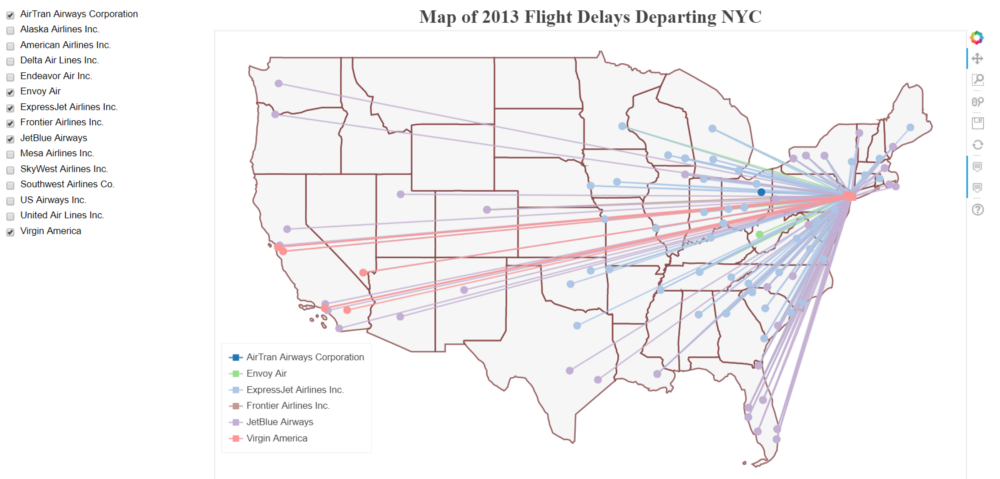def map_tab(map_data, states):
...
def make_dataset(airline_list):
...
return new_src
def make_plot(src):
...
return p

def update(attr, old, new):
...
new_src = make_dataset(airline_list)
src.data.update(new_src.data)

controls = ...
tab = Panel(child = layout, title = 'Flight Map')
return tab



# Put all the tabs into one application
tabs = Tabs(tabs = [tab1, tab2, tab3, tab4, tab5])
# Put the tabs in the current document for display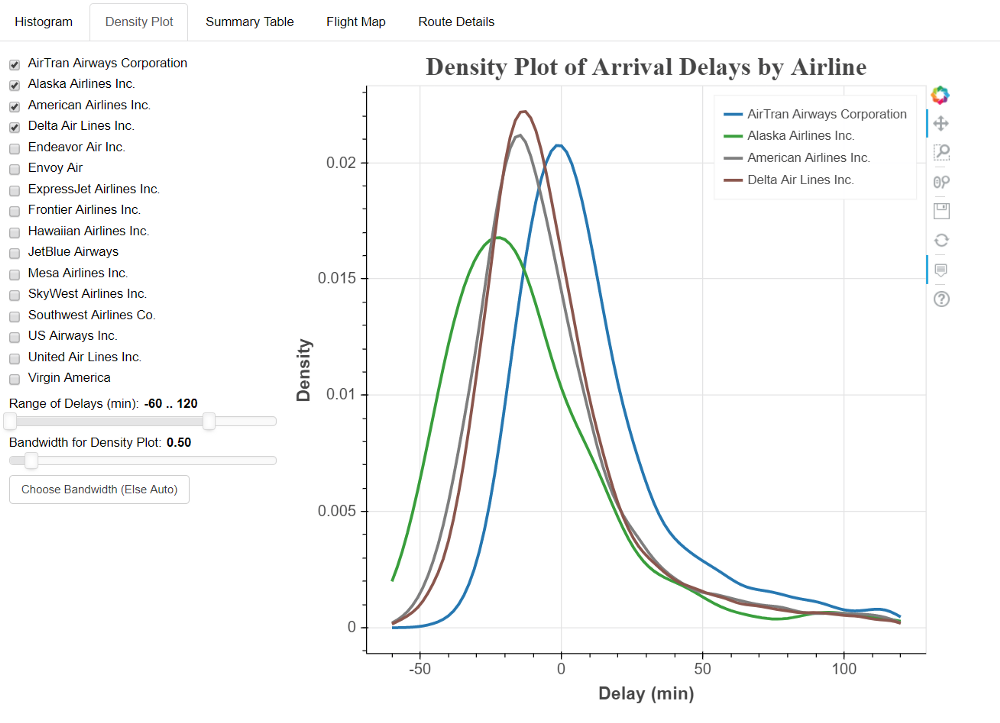### 运行 Bokeh 服务器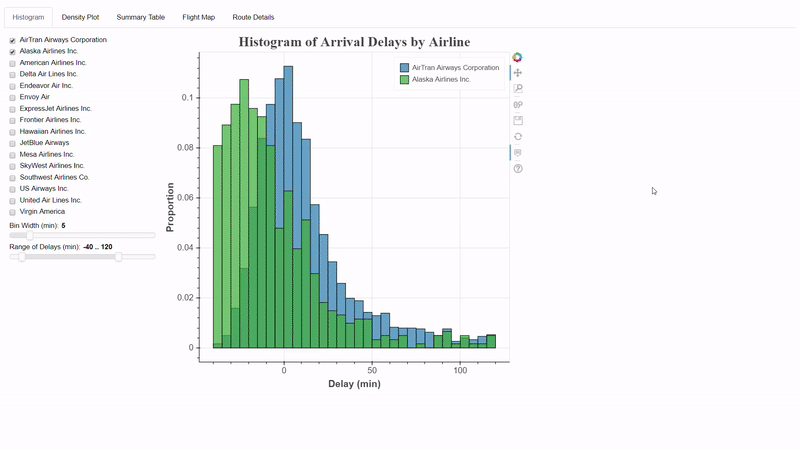### 总结

1. 在开始数据科学任务（Bokeh 或其他任何东西）之前，拥有适当的框架/结构至关重要。 这样，你就不会发现自己迷失在试图查找错误的代码的泥潭中。 此外，一旦我们开发出一个有效的框架，它可以用最少的努力重复使用。
2. 找到一个允许您快速迭代思路的调试工具至关重要。 编写代码 - 查看结果 - 修复错误，这种循环在 Jupyter Notebook 可以实现高效的开发（尤其是对于小规模项目）。
3. Bokeh 中的交互式应用程序将提升您的项目并鼓励用户参与。 仪表板可以是一个独立的探索项目，或突出您已经完成的所有艰难的分析工作！
4. 估计你永远不知道在哪里可以找到你将在工作或辅助项目中使用的下一个工具。 所以，不要害怕尝试新的软件和技术！